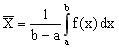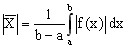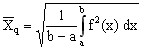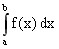# 2D Curve: editing (particular quantities)

## Introduction

All the information pieces concerning the 2D curve are found in its dialogue box:

• the calculation results
• the context of creation

## Values of the curve

The following values are calculated over the definition interval of the 2D curve:

Calculated values definition
Minimal value The minimal value represents the smallest value found on the curve traced in the designed interval.
Maximal value The maximal value stands for the biggest value found on the curve traced in the designed interval.
Average value

The average value of the function f of the curve on the interval [a,b] is defined by formula:Redressed average value

The redressed average of the function f of the curve on the interval [a,b] is defined by formula:The quadratic average of the function f of the curve on the interval [a,b] is defined by formula:Integral The integral along the curveon the interval [a,b] is the value of the domain area limited by the curve representative for the function f, the two verticals x=a and x=b, and the axis of the abscissas.

## Remark on the calculated average values

The calculation of the average values and of the integral is carried out by the method of the trapezes. Indeed, it is possible that certain curves be traced on an interval where the abscissa values are not spaced regularly. That is the reason why the arithmetic average is not used.

## Editing the characteristic quantities

To edit the particular quantities of a 2D Curve, activate the command Edit by one of the following methods:

• from the sheet of the 2D curve by means of a contextual menu
• from the data tree by means of a contextual menu